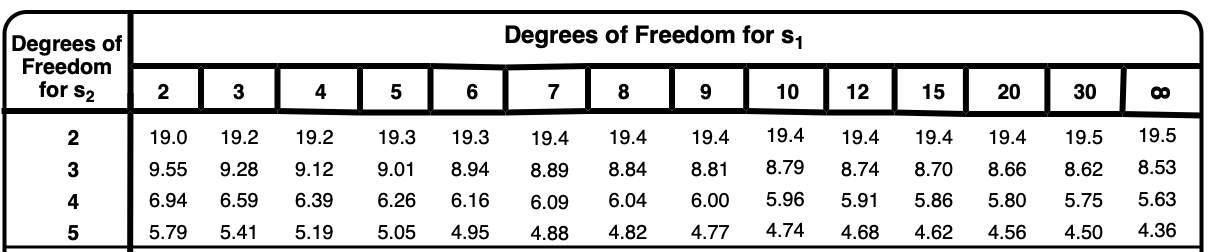Clutch Prep is now a part of Pearson
Ch.4 + 5 - Statistics, Quality Assurance and Calibration MethodsWorksheetSee all chapters

# Analysis of Variance (f-Test)

See all sections
Sections
Mean Evaluation
The Gaussian Distribution
Confidence Intervals
Hypothesis Testing (t-Test)
Analysis of Variance (f-Test)
Detection of Gross Errors

An F-Test is used to compare 2 populations' variances.

###### F-Test

Concept #1: The F-Test allows us to compare the variance of 2 populations by first calculating the quotient###### F-Test Calculations

Example #1: In the process of assessing responsibility for an oil spill, two possible suspects are identified. To differentiate between the two samples of oil, the ratio of the concentration for two polyaromatic hydrocarbons is measured using fluorescence spectroscopy. These values are then compared to the sample obtained from the body of water:

Mean                Standard Deviation         # Samples

Suspect 1                      2.31                              0.073                                    4

Suspect 2                      2.67                              0.092                                    5

Sample                            2.45                              0.088                                    6

From the above results, should there be a concern that any combination of the standard deviation values demonstrates a significant difference?Example #2: Can either (or both) of the suspects be eliminated based on the results of the analysis at the 99% confidence interval?

Example #3: You are measuring the effects of a toxic compound on an enzyme. You expose five (test tubes of cells to 100 µL of a 5 ppm aqueous solution of the toxic compound and mark them as treated, and expose five test tubes of cells to an equal volume of only water and mark them as untreated.  You then measure the enzyme activity of cells in each test tube; enzyme activity is in units of µmol/minute.  The following are the measurements of enzyme activity:

Activity (Treated)                           Activity (Untreated)

Tube            (µmol/min)                     Tube           (µmol/min)

1                            3.25                              1                      5.84

2                            3.98                              2                      6.59

3                            3.79                              3                      5.97

4                            4.15                              4                      6.25

5                            4.04                              5                      6.10

Average:                 3.84                              Average:           6.15

Standard                                                      Standard

Deviation:                0.36                              Deviation:          0.29

Is the variance of the measured enzyme activity of cells exposed to the toxic compound equal to that of cells exposed to water alone?

Example #4: Is the average enzyme activity measured for cells exposed to the toxic compound significantly different (at 95% confidence level) than that measured for cells exposed to water alone?